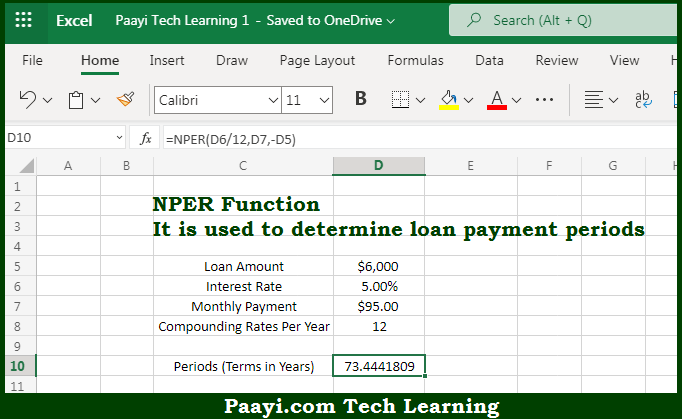# Learn How to Use Microsoft Excel NPER Function

Written by | 0 Comments | 566 Views

In this article, you will learn how to use the Microsoft Excel NPER function and its prime function in Microsoft Excel. You will also get to know the Microsoft Excel NPER function return value and syntax with the help of some examples.

Microsoft Excel NPER Function

The main purpose of the Microsoft Excel NPER function is to get the number of periods for a loan or for an investment. That implies, with the help of the NPER function you can able to return the number of periods for a loan or investment. It should be noted that the NPER function can be used to get the number of payment periods for a loan, where the amount, the interest rate, and the periodic payment amount are given. So, with the help of the NPER function, you can able to get get the number of periods for a loan or for an investment.

Return Value of NPER Function

The return value will be the number of periods.

Syntax of NPER Function

=NPER(rate, pmt, pv, [fv], [type])

Where the arguments:

• rate: This is the interest rate per period.
• pmt: This is the payment made per period.
• pv: This is the present value or the total value of all payments in present.
• fv: This is the future value or a cash balance that can be required after the last payment is made. The default is 0 (Optional).
• type: In case the payments are due, then 0 = end of the period and 1 = beginning of the period. Default is 0 (Optional).

How to Use Microsoft Excel NPER Function?So we know that Microsoft Excel NPER function you can able to get the number of periods for a loan or for an investment. That implies, with the help of the NPER function you can able to return the number of periods for a loan or investment. It should be noted that the NPER function can be used to get the number of payment periods for a loan, where the amount, the interest rate, and the periodic payment amount are given. So, with the help of the NPER function, you can able to get get the number of periods for a loan or for an investment.